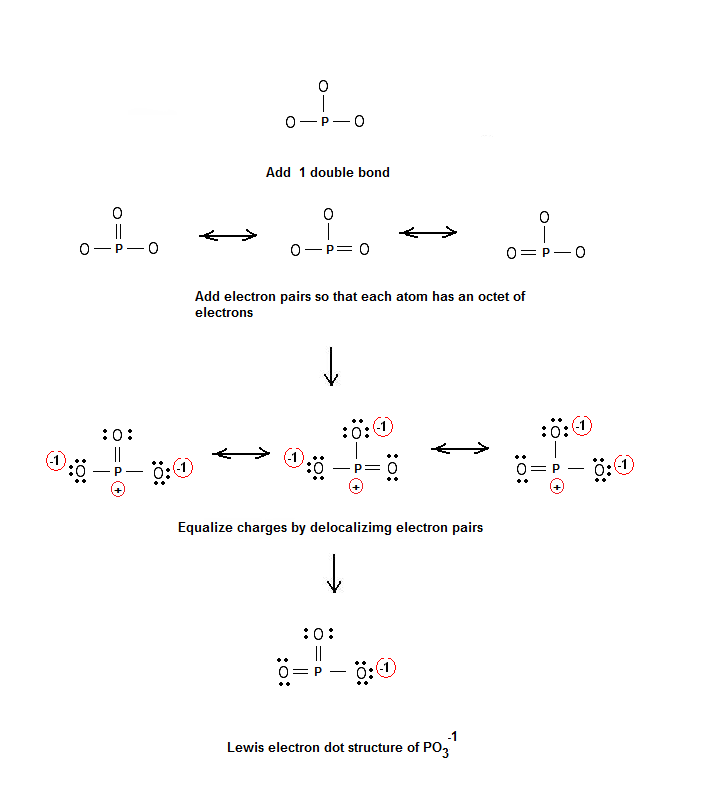Lewis structure of PO3-1 – Simple Method for Lewis Electron Dot Structures | Chemistry Net

# Lewis structure of PO3-1 – Simple Method for Lewis Electron Dot Structures

Simple Method for writing Lewis Electron Dot Structures of the phosphite ion(PO3)-1

# Lewis Structures of the Phosphite Ion (PO3)-1

A simple method for writing Lewis electron dot structures is given in a previous article entitled “Lewis Structures and the Octet Rule”. Several worked examples relevant to this procedure were given in previous posts please see the Sitemap - Table of Contents (Lewis Electron Dot Structures).

Another example for writing Lewis structures following the above procedure is given inthis post.

Let us consider the case of the Lewis structures of phosphite ion PO3-.

Step 1: The central atom will be the P atom since it is the less electronegative. Connect the atoms with single bonds:Step 2: Calculate the # of electrons in π bonds (multiple bonds) using formula (1) in the article entitled “Lewis Structures and the Octet Rule”.:

Where n in this case is 4. Where V = (6 + 6 + 6 + 5) – (-1) = 24 , V is the number of valence electrons of PO3-1. Therefore, P = 6n + 2 – V = 6 * 4 + 2 – 24 = 2

So, there is one double bond in the molecule.

Step 3 & 4: The resonance structures of PO3-1 are as follows:References

1. G.N. Lewis, J.A.C.S, 38, 762-785, (1916)
2. E. C. McGoran, J. Chem. Educ., 68, 19-23 (1991)
3. A.B.P. Lever, J. Chem. Educ., 49, 819-821, (1972)
4. Steven S. Zumdahl, “Chemical Principles” 6th Edition, Houghton Mifflin Company, 2009

Key Terms

Lewis structures of, simple method for writing Lewis electron dot structures, Lewis electron dot structures, electron dot structures